Question

# Given the matrix A and its reduced row echelon form R, answer the following questions. A=...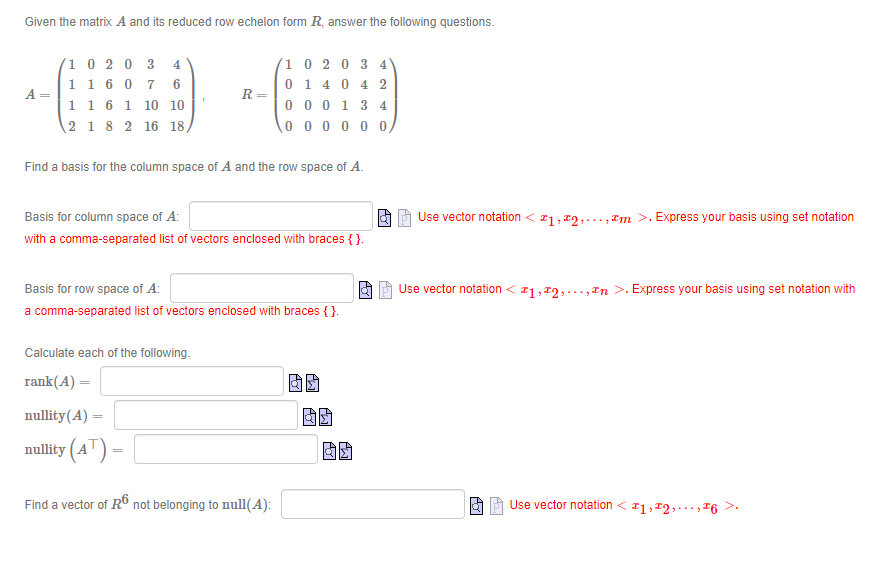Given the matrix A and its reduced row echelon form R, answer the following questions. A= 10 20 3 4 1 1 60 7 6 11 6 1 10 10 2 1 8 2 16 18 R= 1 0 2 0 3 4 0 14 0 4 2 000134 000000 Find a basis for the column space of A and the row space of A. Basis for column space of A: with a comma-separated list of vectors enclosed with braces { } Use vector notation <21,22,..., Im >. Express your basis using set notation Use vector notation<11,12,..., In >. Express your basis using set notation with Basis for row space of A: a comma-separated list of vectors enclosed with braces {}. Calculate each of the following. rank(A) = nullity(A) = nullity (AT) - o Find a vector of R6 not belonging to null(A): Use vector notation <11,12,...,16 >.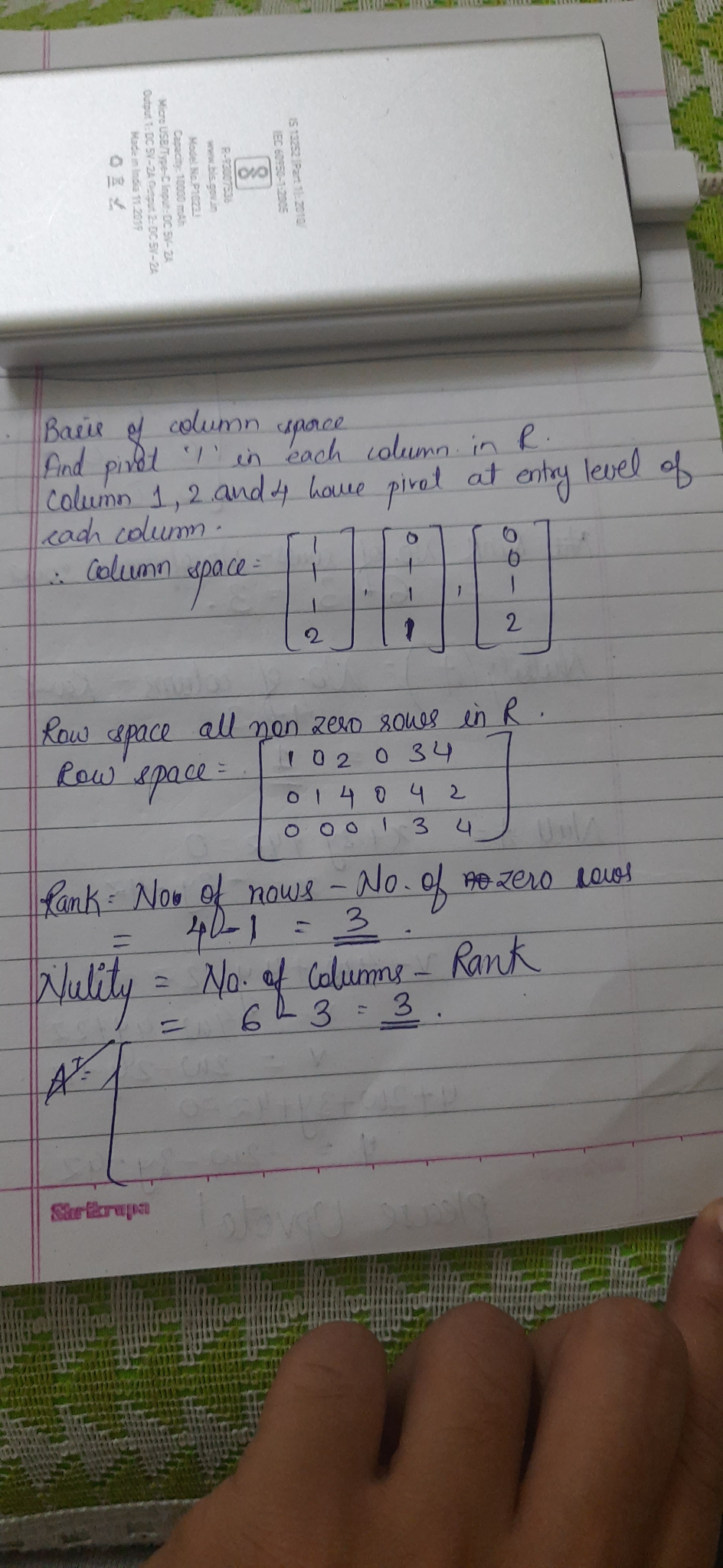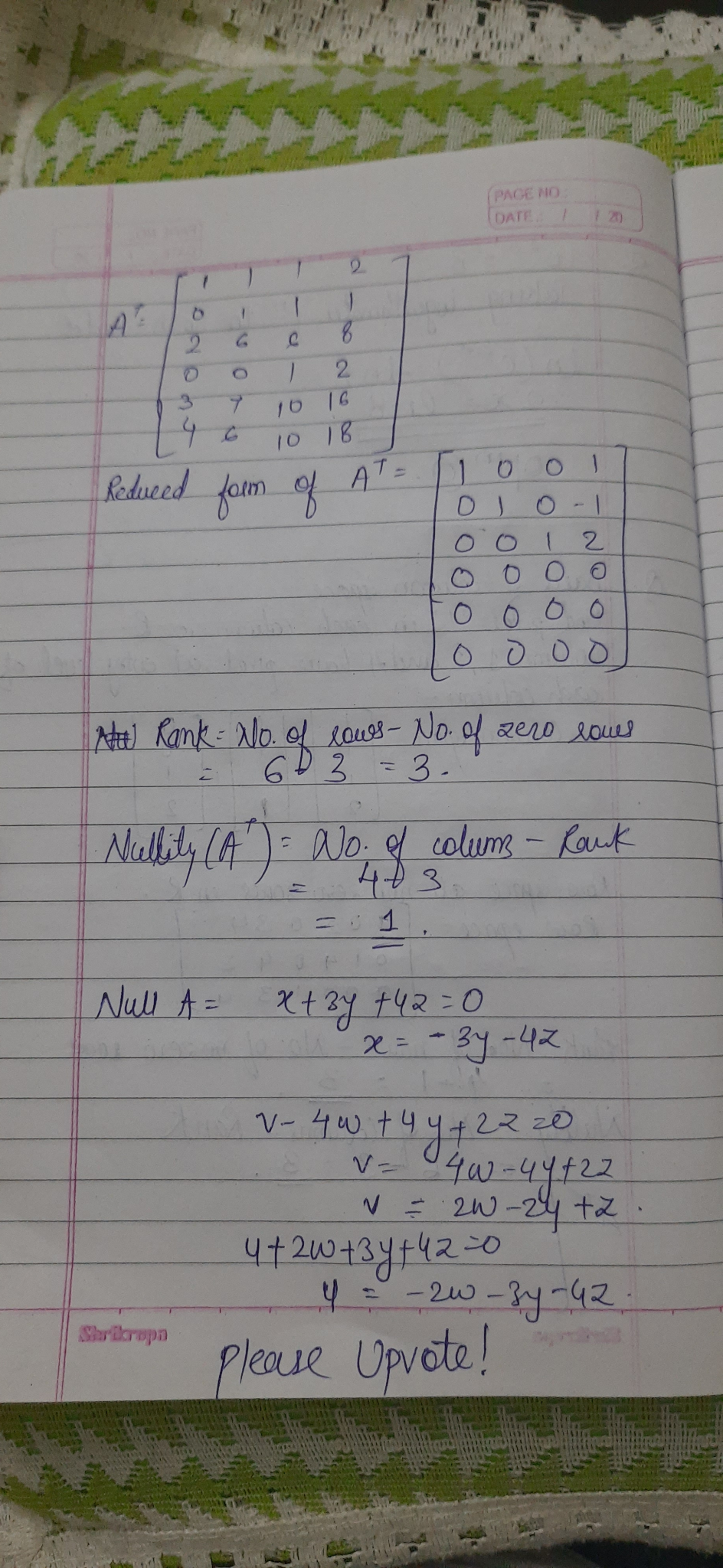#### Earn Coins

Coins can be redeemed for fabulous gifts.

Similar Homework Help Questions
• ### Given the matrix A and its reduced row echelon form R, answer the following questions. A=...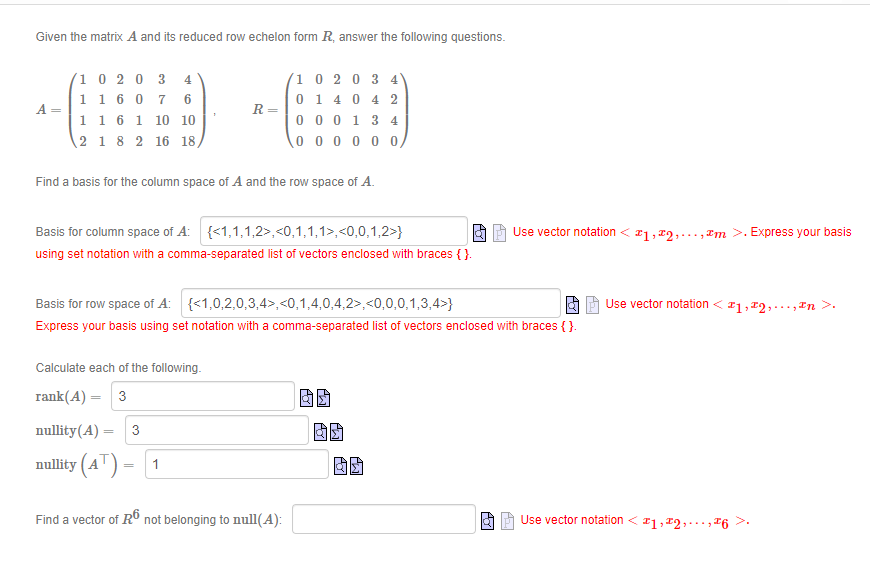Given the matrix A and its reduced row echelon form R, answer the following questions. A= 1 02 03 4 1 1 6 0 7 6 1 1 6 1 10 10 2 1 8 2 16 18 R= (10 20 3 4 01 4 0 4 2 000134 000000 Find a basis for the column space of A and the row space of A. Use vector notation <21,22,..., Im >. Express your basis Basis for column space of A:...

• ### Given the matrix A and its reduced row echelon form R, answer the following questions. 4...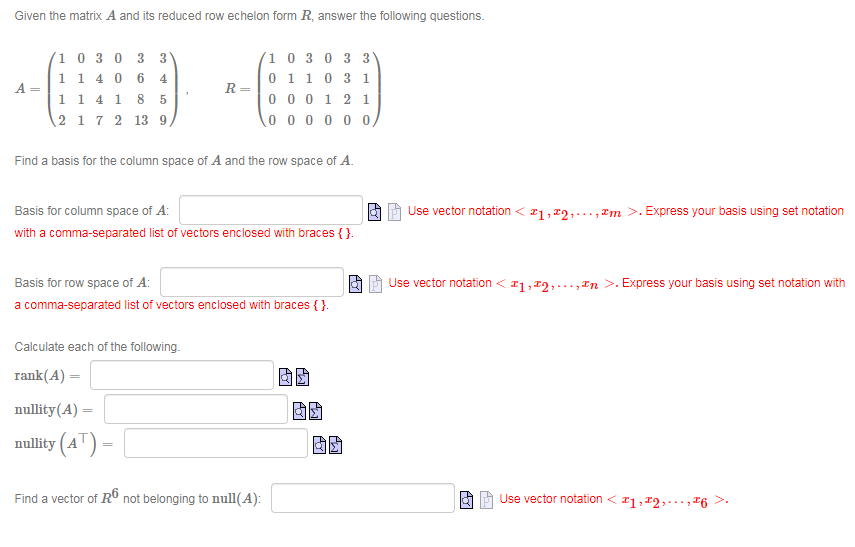Given the matrix A and its reduced row echelon form R, answer the following questions. 4 A 1 0 3 0 3 3 1 1406 1 1 4 1 8 2 1 7 2 13 9 R= 1 0 3 0 3 3 0110 31 000121 1000000 5 Find a basis for the column space of A and the row space of A. Ao Basis for column space of A: with a comma-separated list of vectors enclosed with braces {}....

• ### Question 3 please answer clearly. A matrix A and its reduced row echelon form are given...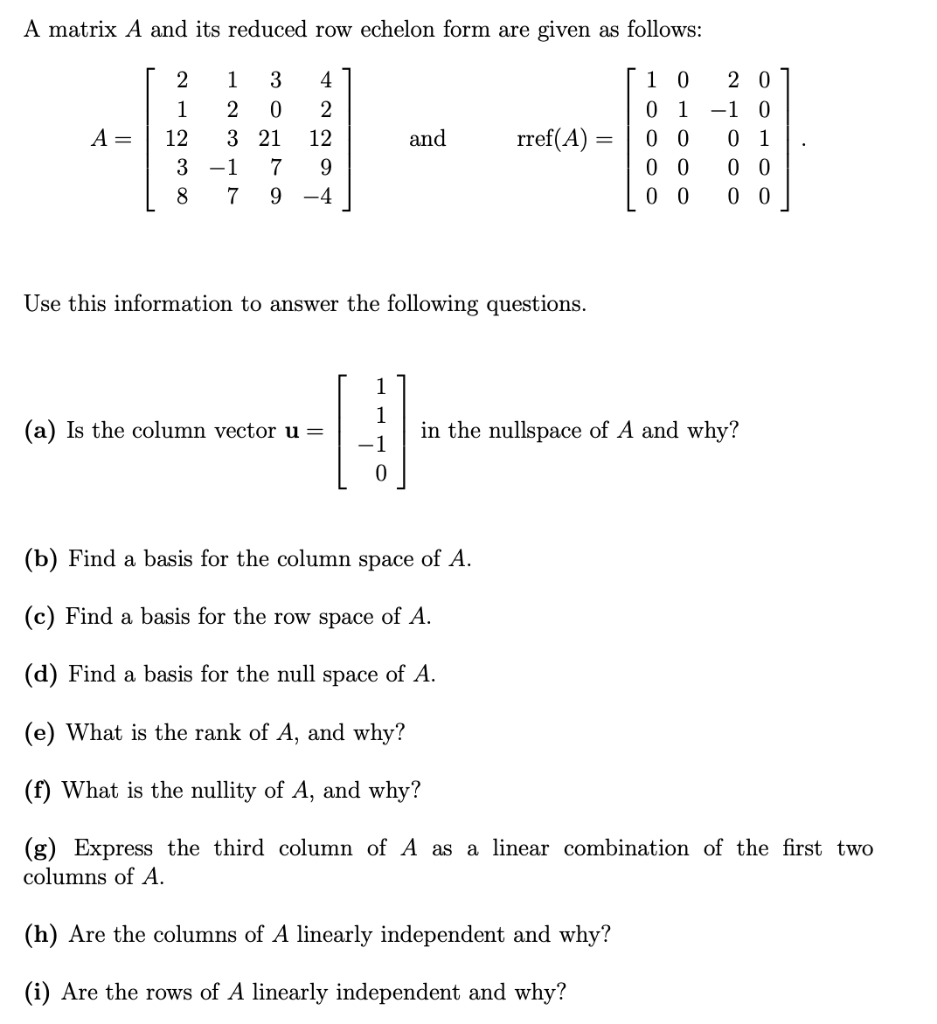Question 3 please answer clearly. A matrix A and its reduced row echelon form are given as follows: [ 2 1 3 41 | 1 2 0 2 A= 3 21 12 | 3 -1 7 9 18 7 9 -4 and rref(A) = [ 1 0 201 0 1 -1 0 0 0 0 1 0 0 0 0 | 0 0 0 0 Use this information to answer the following questions. (a) Is the column vector u= in...

• ### please give the correct answer with explanations, thank you Als a 3 x 4 matrix with...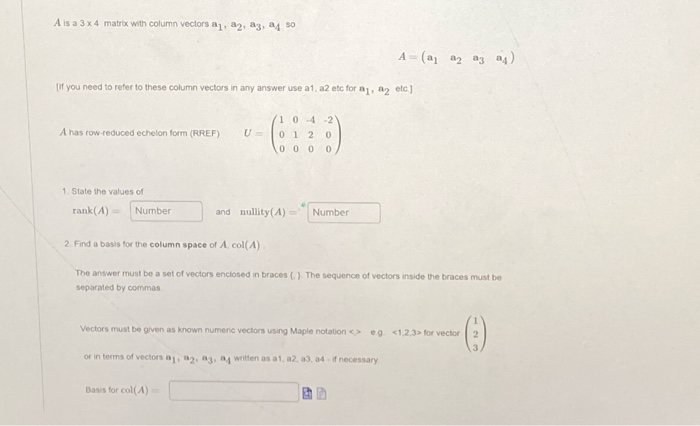please give the correct answer with explanations, thank you Als a 3 x 4 matrix with column vectors a, a, a3, 24 50 A (a a2 a3 24 If you need to refer to these column vectors in any answer use a1 a2 etc for 1, 2 etc.) A has row reduced echelon form (RREF) 1042 0 1 2 0 0 0 0 1. State the values of rank(A) Number and nullity(A) Number 2. Find a basis for the column...

• ### 1. Consider the following matrix and its reduced row echelon form [1 0 3 3 5...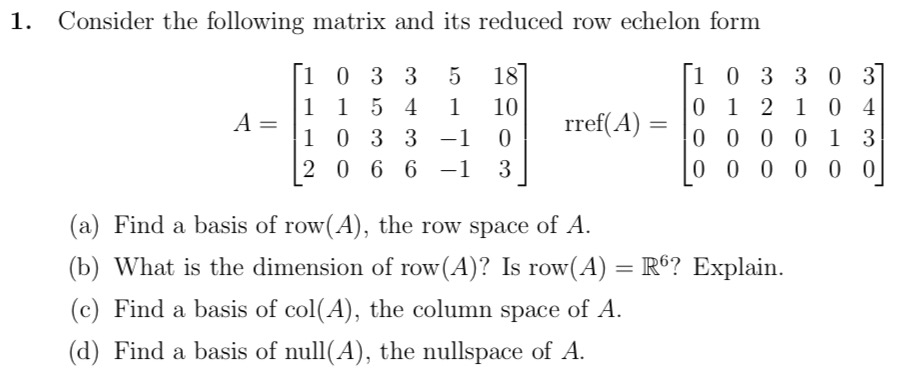1. Consider the following matrix and its reduced row echelon form [1 0 3 3 5 187 [1 0 3 3 0 37 1 1 5 4 1 10 0 1 2 1 0 - A=1 4 1 0 3 3 -1 0 rref(A) = 10 0 0 0 1 3 2 0 6 6 -1 3 | 0 0 0 0 0 0 (a) Find a basis of row(A), the row space of A. (b) What is the dimension...

• ### And its row echelon form (verify ) is given by 1-3 4-2 5 0 01 3- what is the nullity of A without...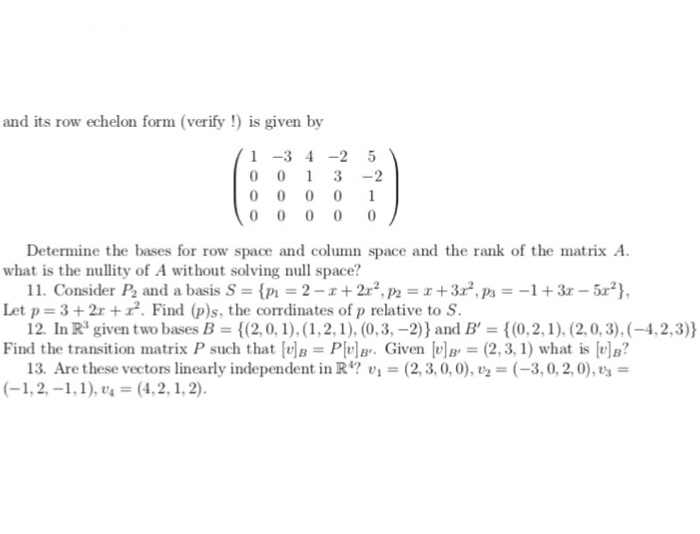Need answer 11~13，as detailed as possible please and its row echelon form (verify ) is given by 1-3 4-2 5 0 01 3- what is the nullity of A without solving null space? Let p 3+2r+. Find (p)s, the corrdinates of p relative to S. Find the transition matrix P such that [tle = Plula.. Given lula, = (2,3, 1) what is lul? Determine the bases for row space and column space and the rank of the matrix A 11....

• ### (a) Use Mathematica to find an echelon form of A. (b) Using your answer to the...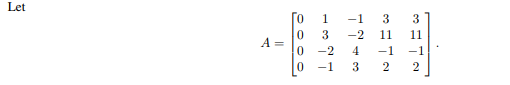(a) Use Mathematica to find an echelon form of A. (b) Using your answer to the previous part, find the rank and nullity of A. (c) Find a basis for the row space of A. (d) Find a basis for the column space of A. (e) Find a basis for the null space of A. Let To 1 3 3 0 -1 3 2 2

• ### Use elementary row operations to reduce the given matrix to row echelon form and reduced row...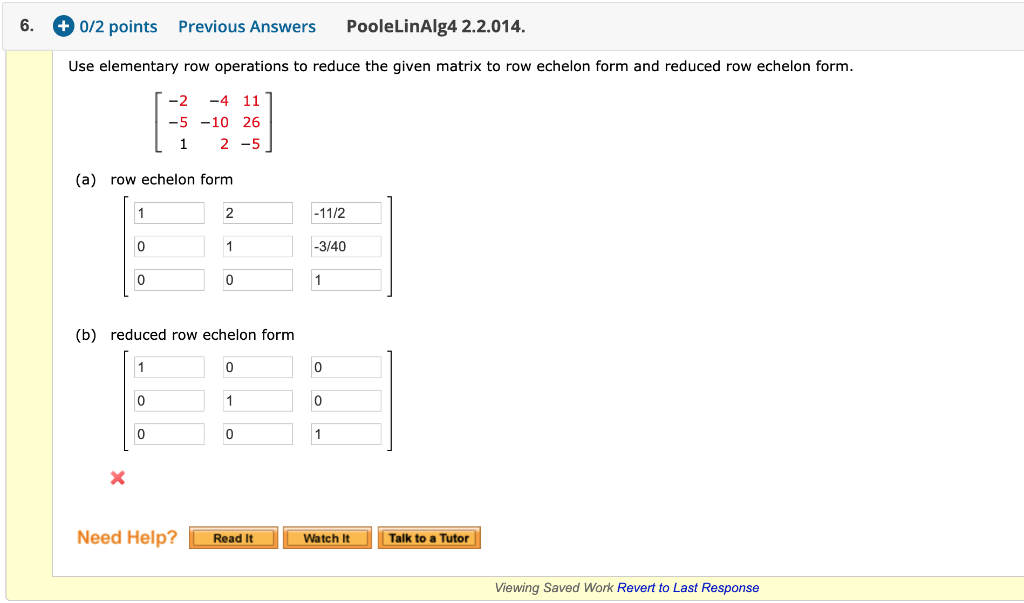Use elementary row operations to reduce the given matrix to row echelon form and reduced row echelon form. Please note when it hits REF and RREF. Thank you! 6. + 0/2 points Previous Answers PooleLinAlg4 2.2.014. Use elementary row operations to reduce the given matrix to row echelon form and reduced row echelon form. [-2 -4 11 | -5 -10 26 Li 2 -5] (a) row echelon form 2 1 -1172 -3/40 0 1 (b) reduced row echelon form 0...

• ### 4. Consider the matrix 0- 3 1 -2 1 4 (a) Use Matlab to determine the reduced row echelon form of A (b) If v, V2, vs, v4...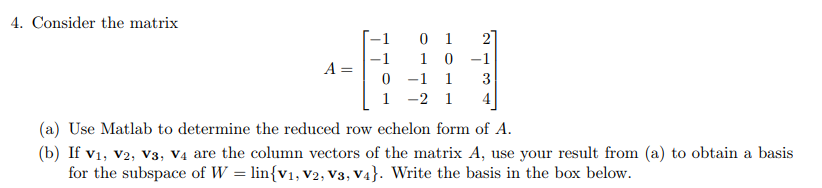4. Consider the matrix 0- 3 1 -2 1 4 (a) Use Matlab to determine the reduced row echelon form of A (b) If v, V2, vs, v4 are the column vectors of the matrix A, use your result from (a) to obtain a basis for the subspace of W-linsv1, V2, V3, V4. Write the basis in the box below 4. Consider the matrix 0- 3 1 -2 1 4 (a) Use Matlab to determine the reduced row echelon form...

• ### [1 -1 0 0 -2 0] 1 4 -4 0 0 -8 0 (1 point) Let...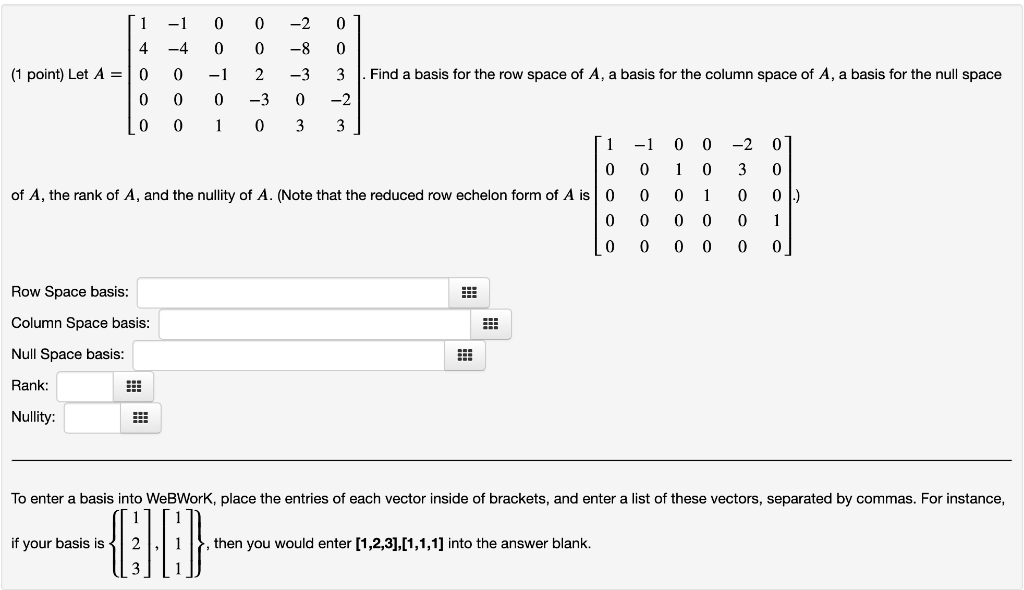[1 -1 0 0 -2 0] 1 4 -4 0 0 -8 0 (1 point) Let A = 10 0 -1 2 -3 3 . Find a basis for the row space of A, a basis for the column space of A, a basis for the null space 0 0 0 -3 0 -2 Lo 0 1 0 3 3] [1 -1 0 0 -2 01 0 0 1 0 3 0 of A, the rank of A, and the...